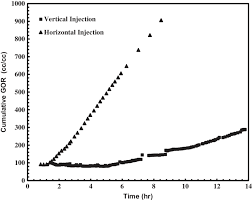## How to Calculate and Solve for Cumulative GOR of Reservoir Fluid Flow | The Calculator EncyclopediaThe image above represents the cumulative GOR.

To compute for the cumulative GOR, two essential parameters are needed and these parameters are cumulative gas production (Gp) and cumulative oil production (Np).

The formula for calculating the cumulative GOR:

Rp = Gp / Np

Where;

Rp = Cumulative GOR
Gp = Cumulative Gas Production
Np = Cumulative Oil Production

Let’s solve an example;
Find the cumulative GOR with a cumulative gas production of 32 and a cumulative oil production of 44.

This implies that;

Gp = Cumulative Gas Production = 32
Np = Cumulative Oil Production = 44

Rp = Gp / Np
Rp = 32 / 44
Rp = 0.72

Therefore, the cumulative GOR is 0.72.

Calculating the Cumulative Gas Production when the Cumulative GOR and the Cumulative Oil Production is Given

Gp = Rp x Np

Where;

Gp = Cumulative Gas Production
Rp = Cumulative GOR
Np = Cumulative Oil Production

Let’s solve an example;
Find the cumulative gas production when the cumulative GOR is 56 and the cumulative oil production is 19.

This implies that;

Rp = Cumulative GOR = 56
Np = Cumulative Oil Production = 19

Gp = Rp x Np
Gp = 56 x 19
Gp = 1064

Therefore, the cumulative gas production is 1064.